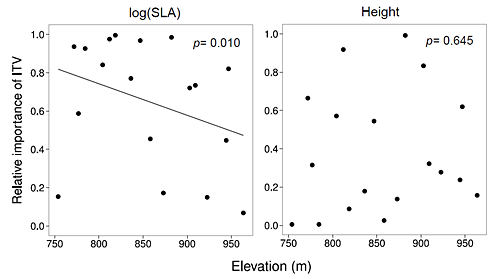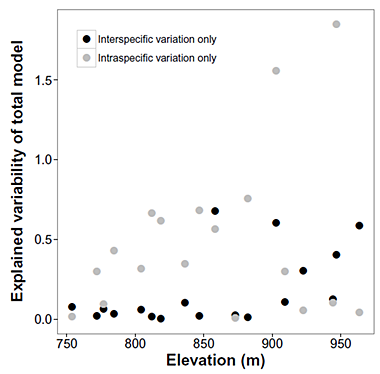Ecological Archives E096-255-A2

Geneviève Lajoie and Mark Vellend. 2015. Understanding context dependence in the contribution of intraspecific variation to community traitenvironment matching. Ecology 96:29122922. http://dx.doi.org/10.1890/15-0156.1

Appendix B. Relative importance of intraspecific variation along the elevational gradient.Fig. B1. Relative importance of ITV to community trait turnover at different points along the elevational gradient. This metric (the y-axis) was calculated within sliding windows of 5 plots along the gradient, for both SLA and height. Each point represents one window of plots, its mid-point along the gradient being represented on the x axis. Statistical significance of permutation tests for a linear relationship between the relative importance of ITV and elevation are indicated in upper right corner of each panel.Fig. B2. Variation in total model variability explained by SPT and ITV components of trait change for SLA along the elevational gradient. Explained variability for each model was calculated as SSRSPT/SSTSPT+ITV and SSRITV/SSTSPT+ITV respectively, within each window of 5 plots. Proportions greater than 1 indicate negative covariance between SPT and ITV drivers of total trait change.